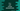# C++ program to sort strings alphabetically## How to sort strings alphabetically in C++:

In this post, we will learn how to sort an array of strings in C++. We will take the strings as inputs from the user and sort them alphabetically and by lenght.

### std::sort

std::sort is a STL library method and we can use it to sort the items in a range. It will by default sort the items in an iterator in non-decreasing order.

It is defined as like below:

``sort(start, end, comp)``

We can provide the start and end positions and also optionally we can use a comparator. By default, the comparator will sort in non-decreasing order.

## Example 1: Use std::sort to sort strings alphabetically:

Let’s use std::sort to sort strings in alphabetic order. We don’t have to provide any comparator. We can simply pass the start and end positions to sort all strings alphabetically.

We will use a vector to store the strings:

``````#include <iostream>
#include <algorithm>
#include <vector>
#include <string>

using namespace std;

int main()
{
vector<string> strArray = {"dog", "cat", "apple", "banana" };

sort(strArray.begin(), strArray.end());

for (int i = 0; i < strArray.size(); i++)
cout << strArray[i] << endl;

return 0;
}``````

Here,

• algorithm header is to use the sort method.
• strArray is the given vector of strings.
• We are using sort to sort all strings in strArray.
• The last for loop is printing the contents of strArray after the sort is done.

If you run this program, it will print:

``````apple
banana
cat
dog``````

Note that it is case sensitive sort. For example, for {“dog”, “Cat”, “apple”, “banana” }, it will put Cat at the top:

``````Cat
apple
banana
dog``````

## Example 2: Use std::sort to sort strings by length:

We can also use std::sort to sort a list of strings by the length of each. We need to use the third comparator function parameter for that. The comparator function can take two strings as its parameter and it needs to return one boolean value.

We can compare the size of each string by using the size() method and return one boolean value.

``````#include <iostream>
#include <algorithm>
#include <vector>
#include <string>

using namespace std;

bool strLengthComparator(string s1, string s2){
return s1.size() < s2.size();
}

int main()
{
vector<string> strArray = {"dog", "Elephant", "apple", "banana" };

sort(strArray.begin(), strArray.end(), strLengthComparator);

for (int i = 0; i < strArray.size(); i++)
cout << strArray[i] << endl;

return 0;
}``````

The function strLengthComparator is used as the string comparator function in this example. We are passing this function as the third parameter to the sort method.

This function compares the size of the strings it is getting as the parameters and returns one true if the length of the first string is smaller than the second string and false otherwise.

If you run this program, it will print the below output:

``````dog
apple
banana
Elephant``````

## Example 3: Use std::sort to sort strings alphabetically in reverse order:

We can use the comparator function to sort the string in reverse order. For example:

``````#include <iostream>
#include <algorithm>
#include <vector>
#include <string>

using namespace std;

bool strReverseComparator(string s1, string s2){
return s1 > s2;
}

int main()
{
vector<string> strArray = {"dog", "elephant", "apple", "banana" , "zebra"};

sort(strArray.begin(), strArray.end(), strReverseComparator);

for (int i = 0; i < strArray.size(); i++)
cout << strArray[i] << endl;

return 0;
}``````

It uses strReverseComparator as the comparator function and that returns true if the first string is greater than the second string.

If you run it, it will print the strings in reverse order as like below:

``````zebra
elephant
dog
banana
apple``````